## Cross Reference Down

#### Kanemitsu, S, Nagasaka, K, Rauzy, G and Shiue, JS (1988). On Benford’s law: the first digit problem. Lecture Notes in Mathematics 1299, pp. 158-169 (eds. Watanabe, S, and Prokhorov, YV).

This work cites the following items of the Benford Online Bibliography:

 Brady, WG (1978). More on Benford’s law. Fibonacci Quarterly 16(1), pp. 51-52.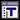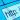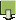Brown, JR and Duncan, RL (1970). Modulo one uniform distribution of the sequence of logarithms of certain recursive sequences. Fibonacci Quarterly 8, pp. 482-486. ISSN/ISBN:0015-0517.Diaconis, P (1977). The Distribution of Leading Digits and Uniform Distribution Mod 1. Annals of Probability 5(1), pp. 72-81. ISSN/ISBN:0091-1798.Duncan, RL (1967). An application of uniform distributions to the Fibonacci numbers. Fibonacci Quarterly 5, pp. 137-140.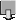Duncan, RL (1969). Note on the initial digit problem. Fibonacci Quarterly 7(5), pp. 474-475.Filipponi, P (1994). Fn and Ln cannot have the same initial digit. Pi Mu Epsilon Journal 10.1, pp. 5-6.Kuipers, L (1969). Remark on a paper by R.L. Duncan concerning the uniform distribution mod 1 of the sequence of the logarithms of the Fibonacci numbers. Fibonacci Quarterly 7, pp. 465-466, 473.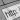Kuipers, L and Niederreiter, H (1974). Uniform Distribution of Sequences. J. Wiley; newer edition - 2006 from Dover. ISSN/ISBN:0486450198.Kuipers, L and Shiue, JS (1973). Remark on a paper by Duncan and Brown on the sequence of logarithms of certain recursive sequences. Fibonacci Quarterly 11(3), pp. 292-294.Nagasaka, K (1984). On Benford's Law. Annals of the Institute of Statistical Mathematics 36(2), pp. 337-352. ISSN/ISBN:0020-3157. DOI:10.1007/BF02481974.Nagasaka, K and Shiue, JS (1986). Benford’s law for linear recurrence sequences. pp 33-54 in: Transcendental numbers and related topics. Proceedings of the Symposium in Kyoto, April 23-25, 1986, by Research Institute for Mathematical Sciences. Kyoto: Kyoto University.Raimi, RA (1976). The First Digit Problem. American Mathematical Monthly 83(7), pp. 521-538. ISSN/ISBN:0002-9890. DOI:10.2307/2319349.Schatte, P (1973). Zur Verteilung der Mantisse in der Gleitkommadarstellung einer Zufallsgröße (Distribution of Mantissa in Floating Point Diagram of Random Variable). Zeitschrift fur Angewandte Mathematik und Mechanik 53(8), 553-565. ISSN/ISBN:0044-2267. DOI:10.1002/zamm.19730530807. GERSchatte, P (1983). On H∞ -summability and the uniform distribution of sequences. Math. Nachr. 113, 237-243. DOI:10.1002/mana.19831130122.Schatte, P (1987). Some estimates of the H∞ -uniform distribution. Monatshefte für Mathematik 103, 233-240.Schatte, P (1988). On the uniform distribution of certain sequences and Benford’s law. Math. Nachr. 136, 271-273. DOI:10.1002/mana.19881360119.Washington, LC (1981). Benford’s law for Fibonacci and Lucas numbers. Fibonacci Quarterly 19, 175-177.Whitney, RE (1972). Initial digits for the sequence of primes. American Mathematical Monthly 79(2), pp. 150-152. ISSN/ISBN:0002-9890.